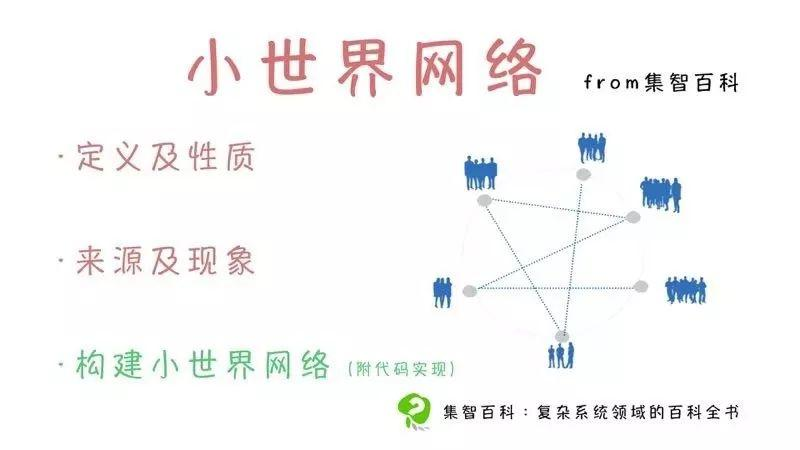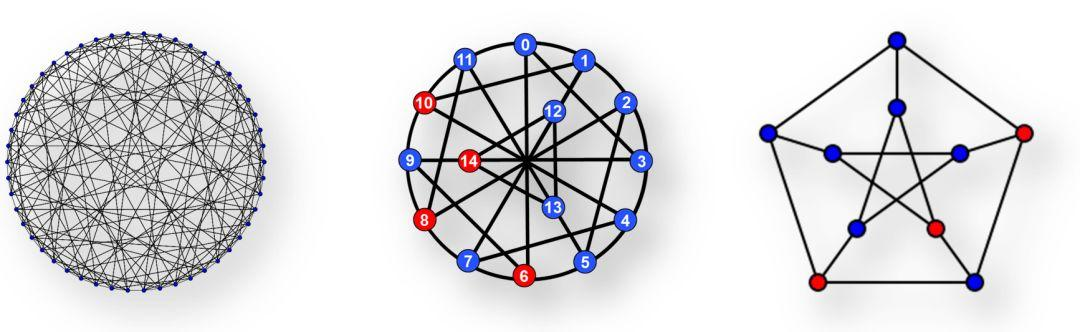Barmpoutis等人给出的从零开始构建小世界网络的另外一种方法，构建了一种平均距离极小且平均集聚度极高的网络。他们提到了一种复杂度恒定的快速算法，以及不同生成图鲁棒性的度量。根据每个网络的应用，可以从一个这样的“超小世界”网络开始，然后重新连接一些边，或者使用几个这样的小网络作为子图，构筑一个更大的图。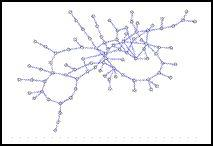`import networkx as nx`
`import matplotlib.pyplot as plt`

`# WS network`
`# 生成20个节点的小世界网络`
`# 每个节点有四个邻居`
`# 每条连边以0.3的概率随机重置链接`
`WS = nx.random_graphs.watts_strogatz_graph(20, 4, 0.3)`
`pos = nx.circular_layout(WS)`
`nx.draw(WS, pos, with_labels = False, node_size = 30)`
`plt.show()`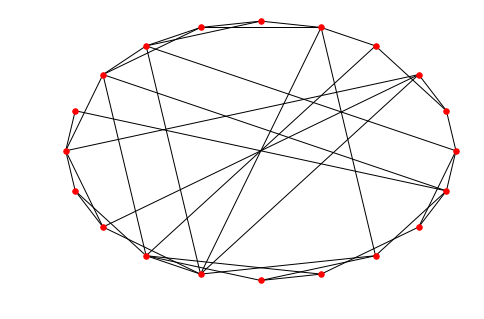`G = nx.Graph()node_num = 20neighbour_num = 4probility = 0.3# 节点与邻居相连for i in range(node_num):     from_node = i      for j in range(neighbour_num):         to_node = (i+j)% node_num - neighbour_num / 2         if to_node >= 0:             to_node += 1         G.add_edge(from_node,to_node)# 连边随机重连edges = copy.deepcopy(G.edges())for edge in edges:        if random.random() <  probility:         G.remove_edge(edge,edge)         ran_edge = np.random.randint(node_num,size=2).tolist()         G.add_edge(ran_edge,ran_edge)# 绘图pos = nx.circular_layout(G)nx.draw(G, pos, with_labels = False, node_size = 30)plt.show()`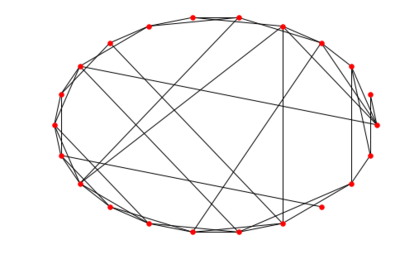http://wiki.swarma.net/index.php/%E5%B0%8F%E4%B8%96%E7%95%8C%E7%BD%91%E7%BB%9C#.E4.BD.BF.E7.94.A8.E5.8E.9F.E7.94.9F.E6.96.B9.E6.B3.95x.E7.94.9F.E6.88.90.E5.B0.8F.E4.B8.96.E7.95.8C.E7.BD.91.E7.BB.9CPC端:

https://campus.swarma.org/gcou=10549# ◆◆◆